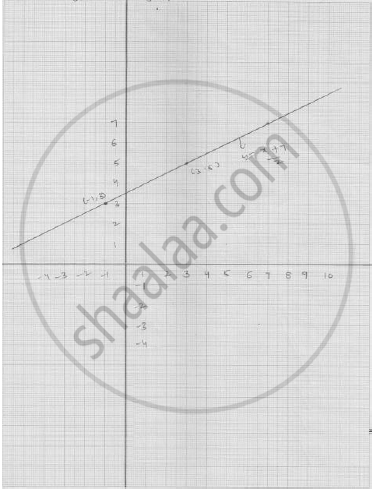# Plot the Points (3, 5) and (− 1, 3) on a Graph Paper and Verify that the Straight Line Passing Through These Points Also Passes Through the Point (1, 4). - Mathematics

Plot the points (3, 5) and (− 1, 3) on a graph paper and verify that the straight line passing
through these points also passes through the point (1, 4).

#### Solution

The points given in the graph:It is clear from the graph the straight lines passes through these points also pass a through
( 1,4 ) .

Concept: Graph of a Linear Equation in Two Variables
Is there an error in this question or solution?

#### APPEARS IN

RD Sharma Mathematics for Class 9
Chapter 7 Linear Equations in Two Variables
Exercise 7.3 | Q 7

Share